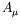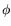Next: Gauge Invariance Up: Klein-Gordon Equation Previous: Orthogonality and Normalization

# Interaction with the Electromagnetic Field

Normally we develop the theory of scalar particles and electromagnetic fields separately. These systems are considered free and independent of each other. Radiative processes arise from the interaction of the two systems. An interaction implies that observable quantities, such as momentum and energy, for instance, can be transfered from one system to the other, thereby giving rise to all kinds of radiation processes. The coupling of the systems can be achieved by postulating the existence of a coupling term in the wave equations which depends on the wave functions of both systems. An obvious restriction which must be satisfied by any theory is the requirement of relativistic invariance of the wave equations. An arbitrary requirement is that the wave equations shall be modified only by terms which are lowest order in the wave functions and contain no derivatives. This means that the interaction term will be linear in the potentialand bilinear (for reasons of relativistic invariance) in the wave function. The final test of the correctness of the interaction term, however, will not be found in such requirements of simplicity but in its agreement with observation.

SubsectionsNext: Gauge Invariance Up: Klein-Gordon Equation Previous: Orthogonality and Normalization
Douglas M. Gingrich (gingrich@ ualberta.ca)
2004-03-18